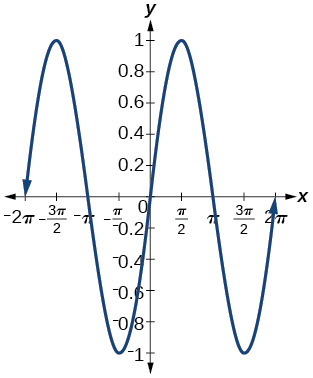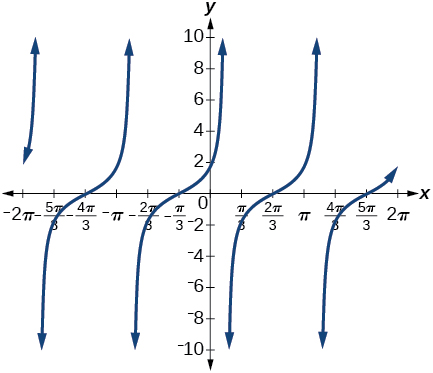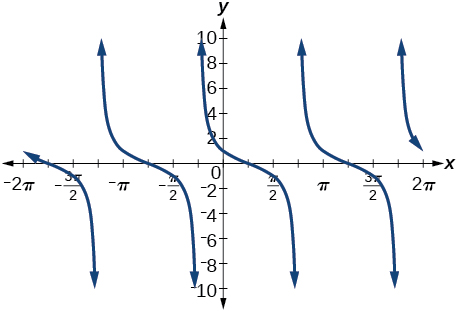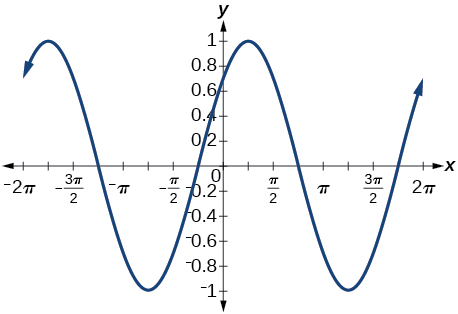# 9.2 Sum and difference identities  (Page 6/6)

 Page 6 / 6

## Verbal

Explain the basis for the cofunction identities and when they apply.

The cofunction identities apply to complementary angles. Viewing the two acute angles of a right triangle, if one of those angles measures $\text{\hspace{0.17em}}x,$ the second angle measures $\text{\hspace{0.17em}}\frac{\pi }{2}-x.\text{\hspace{0.17em}}$ Then $\text{\hspace{0.17em}}\mathrm{sin}x=\mathrm{cos}\left(\frac{\pi }{2}-x\right).\text{\hspace{0.17em}}$ The same holds for the other cofunction identities. The key is that the angles are complementary.

Is there only one way to evaluate $\text{\hspace{0.17em}}\mathrm{cos}\left(\frac{5\pi }{4}\right)?\text{\hspace{0.17em}}$ Explain how to set up the solution in two different ways, and then compute to make sure they give the same answer.

Explain to someone who has forgotten the even-odd properties of sinusoidal functions how the addition and subtraction formulas can determine this characteristic for $\text{\hspace{0.17em}}f\left(x\right)=\mathrm{sin}\left(x\right)\text{\hspace{0.17em}}$ and $\text{\hspace{0.17em}}g\left(x\right)=\mathrm{cos}\left(x\right).\text{\hspace{0.17em}}$ (Hint: $\text{\hspace{0.17em}}0-x=-x$ )

$\mathrm{sin}\left(-x\right)=-\mathrm{sin}x,$ so $\text{\hspace{0.17em}}\mathrm{sin}x\text{\hspace{0.17em}}$ is odd. $\text{\hspace{0.17em}}\mathrm{cos}\left(-x\right)=\mathrm{cos}\left(0-x\right)=\mathrm{cos}x,$ so $\text{\hspace{0.17em}}\mathrm{cos}x\text{\hspace{0.17em}}$ is even.

## Algebraic

For the following exercises, find the exact value.

$\mathrm{cos}\left(\frac{7\pi }{12}\right)$

$\mathrm{cos}\left(\frac{\pi }{12}\right)$

$\frac{\sqrt{2}+\sqrt{6}}{4}$

$\mathrm{sin}\left(\frac{5\pi }{12}\right)$

$\mathrm{sin}\left(\frac{11\pi }{12}\right)$

$\frac{\sqrt{6}-\sqrt{2}}{4}$

$\mathrm{tan}\left(-\frac{\pi }{12}\right)$

$\mathrm{tan}\left(\frac{19\pi }{12}\right)$

$-2-\sqrt{3}$

For the following exercises, rewrite in terms of $\text{\hspace{0.17em}}\mathrm{sin}\text{\hspace{0.17em}}x\text{\hspace{0.17em}}$ and $\text{\hspace{0.17em}}\mathrm{cos}\text{\hspace{0.17em}}x.$

$\mathrm{sin}\left(x+\frac{11\pi }{6}\right)$

$\mathrm{sin}\left(x-\frac{3\pi }{4}\right)$

$-\frac{\sqrt{2}}{2}\mathrm{sin}x-\frac{\sqrt{2}}{2}\mathrm{cos}x$

$\mathrm{cos}\left(x-\frac{5\pi }{6}\right)$

$\mathrm{cos}\left(x+\frac{2\pi }{3}\right)$

$-\frac{1}{2}\mathrm{cos}x-\frac{\sqrt{3}}{2}\mathrm{sin}x$

For the following exercises, simplify the given expression.

$\mathrm{csc}\left(\frac{\pi }{2}-t\right)$

$\mathrm{sec}\left(\frac{\pi }{2}-\theta \right)$

$\mathrm{csc}\theta$

$\mathrm{cot}\left(\frac{\pi }{2}-x\right)$

$\mathrm{tan}\left(\frac{\pi }{2}-x\right)$

$\mathrm{cot}x$

$\mathrm{sin}\left(2x\right)\text{\hspace{0.17em}}\mathrm{cos}\left(5x\right)-\mathrm{sin}\left(5x\right)\text{\hspace{0.17em}}\mathrm{cos}\left(2x\right)$

$\frac{\mathrm{tan}\left(\frac{3}{2}x\right)-\mathrm{tan}\left(\frac{7}{5}x\right)}{1+\mathrm{tan}\left(\frac{3}{2}x\right)\mathrm{tan}\left(\frac{7}{5}x\right)}$

$\mathrm{tan}\left(\frac{x}{10}\right)$

For the following exercises, find the requested information.

Given that $\text{\hspace{0.17em}}\mathrm{sin}\text{\hspace{0.17em}}a=\frac{2}{3}\text{\hspace{0.17em}}$ and $\text{\hspace{0.17em}}\mathrm{cos}\text{\hspace{0.17em}}b=-\frac{1}{4},$ with $\text{\hspace{0.17em}}a\text{\hspace{0.17em}}$ and $\text{\hspace{0.17em}}b\text{\hspace{0.17em}}$ both in the interval $\text{\hspace{0.17em}}\left[\frac{\pi }{2},\pi \right),$ find $\text{\hspace{0.17em}}\mathrm{sin}\left(a+b\right)\text{\hspace{0.17em}}$ and $\text{\hspace{0.17em}}\mathrm{cos}\left(a-b\right).$

Given that $\text{\hspace{0.17em}}\mathrm{sin}\text{\hspace{0.17em}}a=\frac{4}{5},$ and $\text{\hspace{0.17em}}\mathrm{cos}\text{\hspace{0.17em}}b=\frac{1}{3},$ with $\text{\hspace{0.17em}}a\text{\hspace{0.17em}}$ and $\text{\hspace{0.17em}}b\text{\hspace{0.17em}}$ both in the interval $\text{\hspace{0.17em}}\left[0,\frac{\pi }{2}\right),$ find $\text{\hspace{0.17em}}\mathrm{sin}\left(a-b\right)\text{\hspace{0.17em}}$ and $\text{\hspace{0.17em}}\mathrm{cos}\left(a+b\right).$

$\begin{array}{ccccc}\hfill \mathrm{sin}\left(a-b\right)& =& \left(\frac{4}{5}\right)\left(\frac{1}{3}\right)-\left(\frac{3}{5}\right)\left(\frac{2\sqrt{2}}{3}\right)\hfill & =& \frac{4-6\sqrt{2}}{15}\hfill \\ \hfill \mathrm{cos}\left(a+b\right)& =& \left(\frac{3}{5}\right)\left(\frac{1}{3}\right)-\left(\frac{4}{5}\right)\left(\frac{2\sqrt{2}}{3}\right)\hfill & =& \frac{3-8\sqrt{2}}{15}\hfill \end{array}$

For the following exercises, find the exact value of each expression.

$\mathrm{sin}\left({\mathrm{cos}}^{-1}\left(0\right)-{\mathrm{cos}}^{-1}\left(\frac{1}{2}\right)\right)$

$\mathrm{cos}\left({\mathrm{cos}}^{-1}\left(\frac{\sqrt{2}}{2}\right)+{\mathrm{sin}}^{-1}\left(\frac{\sqrt{3}}{2}\right)\right)$

$\frac{\sqrt{2}-\sqrt{6}}{4}$

$\mathrm{tan}\left({\mathrm{sin}}^{-1}\left(\frac{1}{2}\right)-{\mathrm{cos}}^{-1}\left(\frac{1}{2}\right)\right)$

## Graphical

For the following exercises, simplify the expression, and then graph both expressions as functions to verify the graphs are identical. Confirm your answer using a graphing calculator.

$\mathrm{cos}\left(\frac{\pi }{2}-x\right)$

$\mathrm{sin}x$$\mathrm{sin}\left(\pi -x\right)$

$\mathrm{tan}\left(\frac{\pi }{3}+x\right)$

$\mathrm{cot}\left(\frac{\pi }{6}-x\right)$$\mathrm{sin}\left(\frac{\pi }{3}+x\right)$

$\mathrm{tan}\left(\frac{\pi }{4}-x\right)$

$\mathrm{cot}\left(\frac{\pi }{4}+x\right)$$\mathrm{cos}\left(\frac{7\pi }{6}+x\right)$

$\mathrm{sin}\left(\frac{\pi }{4}+x\right)$

$\frac{\mathrm{sin}x}{\sqrt{2}}+\frac{\mathrm{cos}x}{\sqrt{2}}$$\mathrm{cos}\left(\frac{5\pi }{4}+x\right)$

For the following exercises, use a graph to determine whether the functions are the same or different. If they are the same, show why. If they are different, replace the second function with one that is identical to the first. (Hint: think $\text{\hspace{0.17em}}2x=x+x.$ )

$f\left(x\right)=\mathrm{sin}\left(4x\right)-\mathrm{sin}\left(3x\right)\mathrm{cos}\text{\hspace{0.17em}}x,g\left(x\right)=\mathrm{sin}\text{\hspace{0.17em}}x\text{\hspace{0.17em}}\mathrm{cos}\left(3x\right)$

They are the same.

$f\left(x\right)=\mathrm{cos}\left(4x\right)+\mathrm{sin}\text{\hspace{0.17em}}x\text{\hspace{0.17em}}\mathrm{sin}\left(3x\right),g\left(x\right)=-\mathrm{cos}\text{\hspace{0.17em}}x\text{\hspace{0.17em}}\mathrm{cos}\left(3x\right)$

$f\left(x\right)=\mathrm{sin}\left(3x\right)\mathrm{cos}\left(6x\right),g\left(x\right)=-\mathrm{sin}\left(3x\right)\mathrm{cos}\left(6x\right)$

They are the different, try $\text{\hspace{0.17em}}g\left(x\right)=\mathrm{sin}\left(9x\right)-\mathrm{cos}\left(3x\right)\mathrm{sin}\left(6x\right).$

$f\left(x\right)=\mathrm{sin}\left(4x\right),g\left(x\right)=\mathrm{sin}\left(5x\right)\mathrm{cos}\text{\hspace{0.17em}}x-\mathrm{cos}\left(5x\right)\mathrm{sin}\text{\hspace{0.17em}}x$

$f\left(x\right)=\mathrm{sin}\left(2x\right),g\left(x\right)=2\text{\hspace{0.17em}}\mathrm{sin}\text{\hspace{0.17em}}x\text{\hspace{0.17em}}\mathrm{cos}\text{\hspace{0.17em}}x$

They are the same.

$f\left(\theta \right)=\mathrm{cos}\left(2\theta \right),g\left(\theta \right)={\mathrm{cos}}^{2}\theta -{\mathrm{sin}}^{2}\theta$

$f\left(\theta \right)=\mathrm{tan}\left(2\theta \right),g\left(\theta \right)=\frac{\mathrm{tan}\text{\hspace{0.17em}}\theta }{1+{\mathrm{tan}}^{2}\theta }$

They are the different, try $\text{\hspace{0.17em}}g\left(\theta \right)=\frac{2\text{\hspace{0.17em}}\mathrm{tan}\theta }{1-{\mathrm{tan}}^{2}\theta }.$

$f\left(x\right)=\mathrm{sin}\left(3x\right)\mathrm{sin}\text{\hspace{0.17em}}x,g\left(x\right)={\mathrm{sin}}^{2}\left(2x\right){\mathrm{cos}}^{2}x-{\mathrm{cos}}^{2}\left(2x\right){\mathrm{sin}}^{2}x$

$f\left(x\right)=\mathrm{tan}\left(-x\right),g\left(x\right)=\frac{\mathrm{tan}\text{\hspace{0.17em}}x-\mathrm{tan}\left(2x\right)}{1-\mathrm{tan}\text{\hspace{0.17em}}x\text{\hspace{0.17em}}\mathrm{tan}\left(2x\right)}$

They are different, try $\text{\hspace{0.17em}}g\left(x\right)=\frac{\mathrm{tan}x-\mathrm{tan}\left(2x\right)}{1+\mathrm{tan}x\mathrm{tan}\left(2x\right)}.$

## Technology

For the following exercises, find the exact value algebraically, and then confirm the answer with a calculator to the fourth decimal point.

$\mathrm{sin}\left(75°\right)$

$\mathrm{sin}\left(195°\right)$

$\mathrm{cos}\left(165°\right)$

$\mathrm{cos}\left(345°\right)$

$\frac{1+\sqrt{3}}{2\sqrt{2}},$ or 0.9659

$\mathrm{tan}\left(-15°\right)$

## Extensions

For the following exercises, prove the identities provided.

$\mathrm{tan}\left(x+\frac{\pi }{4}\right)=\frac{\mathrm{tan}\text{\hspace{0.17em}}x+1}{1-\mathrm{tan}\text{\hspace{0.17em}}x}$

$\begin{array}{ccc}\hfill \mathrm{tan}\left(x+\frac{\pi }{4}\right)& =& \\ \hfill \frac{\mathrm{tan}x+\mathrm{tan}\left(\frac{\pi }{4}\right)}{1-\mathrm{tan}x\mathrm{tan}\left(\frac{\pi }{4}\right)}& =& \\ \hfill \frac{\mathrm{tan}x+1}{1-\mathrm{tan}x\left(1\right)}& =& \frac{\mathrm{tan}x+1}{1-\mathrm{tan}x}\hfill \end{array}$

$\frac{\mathrm{tan}\left(a+b\right)}{\mathrm{tan}\left(a-b\right)}=\frac{\mathrm{sin}\text{\hspace{0.17em}}a\text{\hspace{0.17em}}\mathrm{cos}\text{\hspace{0.17em}}a+\mathrm{sin}\text{\hspace{0.17em}}b\text{\hspace{0.17em}}\mathrm{cos}\text{\hspace{0.17em}}b}{\mathrm{sin}\text{\hspace{0.17em}}a\text{\hspace{0.17em}}\mathrm{cos}\text{\hspace{0.17em}}a-\mathrm{sin}\text{\hspace{0.17em}}b\text{\hspace{0.17em}}\mathrm{cos}\text{\hspace{0.17em}}b}$

$\frac{\mathrm{cos}\left(a+b\right)}{\mathrm{cos}\text{\hspace{0.17em}}a\text{\hspace{0.17em}}\mathrm{cos}\text{\hspace{0.17em}}b}=1-\mathrm{tan}\text{\hspace{0.17em}}a\text{\hspace{0.17em}}\mathrm{tan}\text{\hspace{0.17em}}b$

$\begin{array}{ccc}\hfill \frac{\mathrm{cos}\left(a+b\right)}{\mathrm{cos}a\mathrm{cos}b}& =& \\ \hfill \frac{\mathrm{cos}a\mathrm{cos}b}{\mathrm{cos}a\mathrm{cos}b}-\frac{\mathrm{sin}a\mathrm{sin}b}{\mathrm{cos}a\mathrm{cos}b}& =& 1-\mathrm{tan}a\mathrm{tan}b\hfill \end{array}$

$\mathrm{cos}\left(x+y\right)\mathrm{cos}\left(x-y\right)={\mathrm{cos}}^{2}x-{\mathrm{sin}}^{2}y$

$\frac{\mathrm{cos}\left(x+h\right)-\mathrm{cos}\text{\hspace{0.17em}}x}{h}=\mathrm{cos}\text{\hspace{0.17em}}x\frac{\mathrm{cos}\text{\hspace{0.17em}}h-1}{h}-\mathrm{sin}\text{\hspace{0.17em}}x\frac{\mathrm{sin}\text{\hspace{0.17em}}h}{h}$

$\begin{array}{ccc}\hfill \frac{\mathrm{cos}\left(x+h\right)-\mathrm{cos}x}{h}& =& \\ \hfill \frac{\mathrm{cos}x\mathrm{cosh}-\mathrm{sin}x\mathrm{sinh}-\mathrm{cos}x}{h}& =& \\ \hfill \frac{\mathrm{cos}x\left(\mathrm{cosh}-1\right)-\mathrm{sin}x\mathrm{sinh}}{h}& =& \mathrm{cos}x\frac{\mathrm{cos}h-1}{h}-\mathrm{sin}x\frac{\mathrm{sin}h}{h}\hfill \end{array}$

For the following exercises, prove or disprove the statements.

$\mathrm{tan}\left(u+v\right)=\frac{\mathrm{tan}\text{\hspace{0.17em}}u+\mathrm{tan}\text{\hspace{0.17em}}v}{1-\mathrm{tan}\text{\hspace{0.17em}}u\text{\hspace{0.17em}}\mathrm{tan}\text{\hspace{0.17em}}v}$

$\mathrm{tan}\left(u-v\right)=\frac{\mathrm{tan}\text{\hspace{0.17em}}u-\mathrm{tan}\text{\hspace{0.17em}}v}{1+\mathrm{tan}\text{\hspace{0.17em}}u\text{\hspace{0.17em}}\mathrm{tan}\text{\hspace{0.17em}}v}$

True

$\frac{\mathrm{tan}\left(x+y\right)}{1+\mathrm{tan}\text{\hspace{0.17em}}x\text{\hspace{0.17em}}\mathrm{tan}\text{\hspace{0.17em}}x}=\frac{\mathrm{tan}\text{\hspace{0.17em}}x+\mathrm{tan}\text{\hspace{0.17em}}y}{1-{\mathrm{tan}}^{2}x\text{\hspace{0.17em}}{\mathrm{tan}}^{2}y}$

If $\text{\hspace{0.17em}}\alpha ,\beta ,$ and $\text{\hspace{0.17em}}\gamma \text{\hspace{0.17em}}$ are angles in the same triangle, then prove or disprove $\text{\hspace{0.17em}}\mathrm{sin}\left(\alpha +\beta \right)=\mathrm{sin}\text{\hspace{0.17em}}\gamma .$

True. Note that $\text{\hspace{0.17em}}\mathrm{sin}\left(\alpha +\beta \right)=\mathrm{sin}\left(\pi -\gamma \right)\text{\hspace{0.17em}}$ and expand the right hand side.

If $\text{\hspace{0.17em}}\alpha ,\beta ,$ and $\text{\hspace{0.17em}}y\text{\hspace{0.17em}}$ are angles in the same triangle, then prove or disprove $\text{\hspace{0.17em}}\mathrm{tan}\text{\hspace{0.17em}}\alpha +\mathrm{tan}\text{\hspace{0.17em}}\beta +\mathrm{tan}\text{\hspace{0.17em}}\gamma =\mathrm{tan}\text{\hspace{0.17em}}\alpha \text{\hspace{0.17em}}\mathrm{tan}\text{\hspace{0.17em}}\beta \text{\hspace{0.17em}}\mathrm{tan}\text{\hspace{0.17em}}\gamma$

#### Questions & Answers

write down the polynomial function with root 1/3,2,-3 with solution
if A and B are subspaces of V prove that (A+B)/B=A/(A-B)
write down the value of each of the following in surd form a)cos(-65°) b)sin(-180°)c)tan(225°)d)tan(135°)
Prove that (sinA/1-cosA - 1-cosA/sinA) (cosA/1-sinA - 1-sinA/cosA) = 4
what is the answer to dividing negative index
In a triangle ABC prove that. (b+c)cosA+(c+a)cosB+(a+b)cisC=a+b+c.
give me the waec 2019 questions
the polar co-ordinate of the point (-1, -1)
prove the identites sin x ( 1+ tan x )+ cos x ( 1+ cot x )= sec x + cosec x
tanh`(x-iy) =A+iB, find A and B
B=Ai-itan(hx-hiy)
Rukmini
what is the addition of 101011 with 101010
If those numbers are binary, it's 1010101. If they are base 10, it's 202021.
Jack
extra power 4 minus 5 x cube + 7 x square minus 5 x + 1 equal to zero
the gradient function of a curve is 2x+4 and the curve passes through point (1,4) find the equation of the curve
1+cos²A/cos²A=2cosec²A-1
test for convergence the series 1+x/2+2!/9x3ByByBy Nick SwainByByByBy